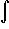#Interactive Real Analysis

Next | Previous | Glossary | Map

## 7.4. Lebesgue Integral

### Example 7.4.11(b): Properties of the Lebesgue Integral

If f is Lebesgue integrable over E and Af(x)B then show that
A m(E)E f(x) dxB m(E)
We should add the condition that m(E) is finite before we start ...

Now define the simple functions

s(x) = A XE(x)
S(x) = B XE(x)
Because f is bounded by A and B we have
s(x)f(x)S(x)
But then the result follows easily from the properties of the Lebesgue integral:
A m(E) =s(x) dxE f(x) dxS(x) dx = B m(E)
Next | Previous | Glossary | Map